# Design of Sewer Notes | Study Environmental Engineering - Civil Engineering (CE)

## Civil Engineering (CE): Design of Sewer Notes | Study Environmental Engineering - Civil Engineering (CE)

The document Design of Sewer Notes | Study Environmental Engineering - Civil Engineering (CE) is a part of the Civil Engineering (CE) Course Environmental Engineering.
All you need of Civil Engineering (CE) at this link: Civil Engineering (CE)

Hydraulic formulas for Determining Flow Velocities in Sewers and Drains

(i) Chezy’s Formula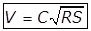Where,
C = Chezy’s constant
R = Hydraulic mean depth or Hydraulic Radius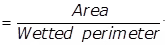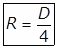for circular sewer of dia D (in Running full)
Discharge,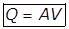Where,
A = Flow area of cross-section.
(a) Kutters Formula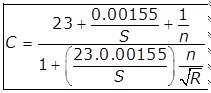Where,
n = Rugosity coefficient
S = Bed slope
(b) Bazin formula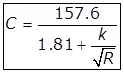Where, k = Bazin constant.
(ii) Manning’s Formula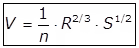(iii) Crimp and Burge’s Formula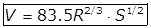This formula is close to Manning’s formula for all those sewer materials for which,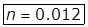(iv) William Hazen’s Formula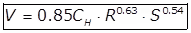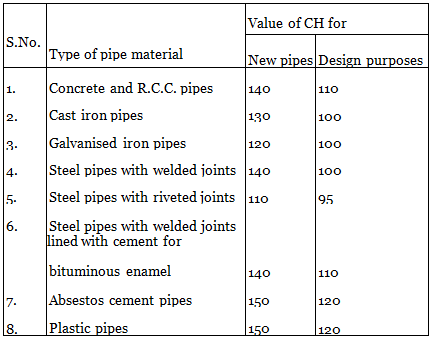Shields Expression for Self Cleansing Velocity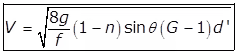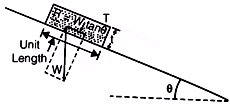where,
f = Friction Factor
n = Porosity
sin θ ~ tan θ - Slope
⇒ θ = tan-1 (Slope)
G = Specific gravity of solid
d = Avg size of solid.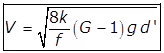where, k = (1 - n) sin θ

Hydraulic Characteristics of Circular Sewer Free Board Provision
(i) Depth of partial flow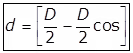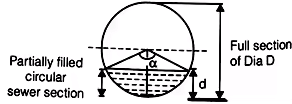where,
α = Central angle in degree
d = Depth of partial flow
D  Dia of full section
(ii) Proportionate depth, (d/D)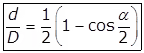(iii) Proportionate depth, (a/A)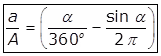Where,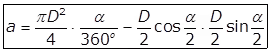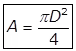(iv) Proportionate perimeter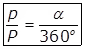Where,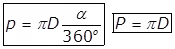(v) Proportionate Hydraulic Mean Depth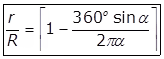(vi) Proportionate Velocity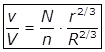(a) If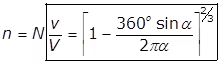(vii) Proportionate Discharge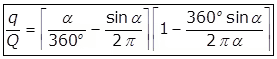(viii) For two sewers whose degree of cleansing is same.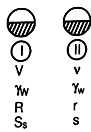i.e.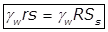(a) Ss/S = R/r
(b) Vs/V = N/n · (r/R)1/6
(c) qs/Q = N/n ·(a/A) (r/R)1/6

•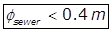design to run 1/2 full of Q design.
•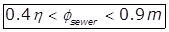design to run 2/3 full of Q design.
•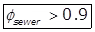design to run 3/4 full of design.

where, ϕ Dia of sewer.
Qdesign = Design discharge.
Sewer is designed for Maximum Hourly Discharge
(i) Maximum hourly discharge
= 1.5 (Maximum daily discharge)
(ii) Maximum daily discharge
= 2.0 (Avg daily discharge)
(iii) Maximum hourly discharge
= 3.0 (Avg daily discharge)Estimation of Peak Drainage Discharge
(i)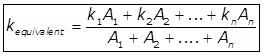Where, k = Runoff/rainoff
Suffix 1,2, … n used for k is runoff of 1,2, ….n.
A = Area.
(ii)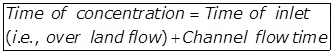(iii)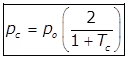Where,
Maximum rainfall intensity of a particular frequency having duration equal to time of concentration.
po = (Point rainfall intensity of same frequency as PC) × Area dispersion factor. (cm/hr)
Tc = Time of concentration in hr.
(iv)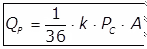where,
QP = Peak discharge in m3/sec.
A = Catchment area in (Hectare)
Critical rainfall in (cm/hour)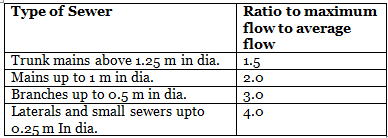The document Design of Sewer Notes | Study Environmental Engineering - Civil Engineering (CE) is a part of the Civil Engineering (CE) Course Environmental Engineering.
All you need of Civil Engineering (CE) at this link: Civil Engineering (CE)Use Code STAYHOME200 and get INR 200 additional OFF

## Environmental Engineering

14 videos|74 docs|70 tests

Track your progress, build streaks, highlight & save important lessons and more!

,

,

,

,

,

,

,

,

,

,

,

,

,

,

,

,

,

,

,

,

,

;